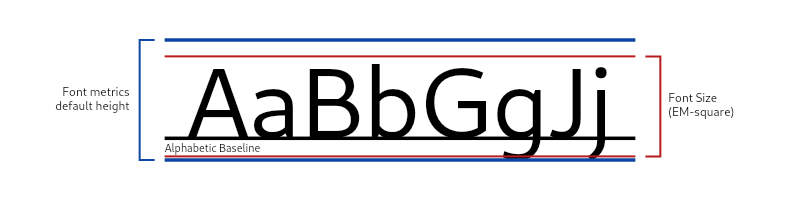# height property

double height
final

The multiple of fontSize to multiply the ascent and descent by where `ascent + descent = fontSize`.

Ascent is the spacing above the baseline and descent is the spacing below the baseline.

When height is omitted or null, then the font defined ascent and descent will be used. The font's combined ascent and descent may be taller or shorter than the fontSize. When height is provided, the line's EM-square ascent and descent (which sums to fontSize) will be scaled by height to achieve a final line height of `fontSize * height + fontSize * leading` logical pixels. The following diagram illustrates the differences between the font metrics defined height and the EM-square height:The height will impact the spacing above and below the baseline differently depending on the ratios between the font's ascent and descent. This property is separate from the leading multiplier, which is controlled through leading.

The ratio of ascent:descent with height specified is the same as the font metrics defined ascent:descent ratio when height is null or omitted.

See TextStyle.height, which works in a similar manner.

The default height is null.

## Implementation

``````final double height

``````